Product Search Results

30 out of 54 item(s)

List View

1.Cal Ver LQ Blood Gas

Order Number: K727M-5
2.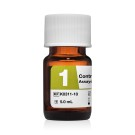Control FD Assayed Chemistry, Level 1

Order Number: K0311-10
3.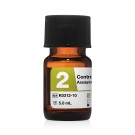Control FD Assayed Chemistry, Level 2

Order Number: K0312-10
4.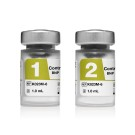Control FD BNP

Order Number: K023M-6
5.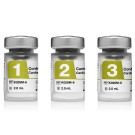Control FD Cardiac Markers

Order Number: K026M-6
6.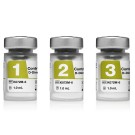Control FD D-Dimer

Order Number: K072M-6
7.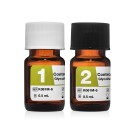Control FD Glycohemoglobin A1c

Order Number: K061M-6
8.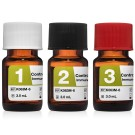Control FD Immunoassay

Order Number: K063M-6
9.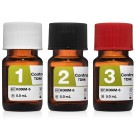Control FD TDM

Order Number: K068M-6
10.Control FD Tumor Markers

Order Number: K070M-6
11.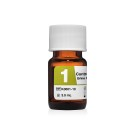Control FD Urine Chemistry, Level 1

Order Number: K0661-10
12.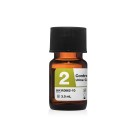Control FD Urine Chemistry, Level 2

Order Number: K0662-10
13.Control LQ COVID-19 Antibodies

Order Number: K077M-6
14.Control LQ Galectin-3

Order Number: K081M-6
15.Control LQ Glycohemoglobin A1c

Order Number: K067M-8
16.Control LQ Homocysteine

Order Number: K020M-6
17.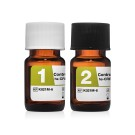Control LQ hs-CRP

Order Number: K021M-6
18.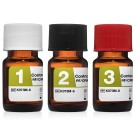Control LQ RF/CRP

Order Number: K073M-6
19.Control LQ Serum Protein

Order Number: K074M-6
20.Control LQ Vitamin D

Order Number: K075M-6
21.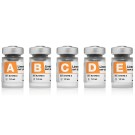Linearity FD BNP (Extended)

Order Number: K737M-5
22.Linearity FD BNP (High)

Order Number: K713M-5
23.Linearity FD BNP (Low)

Order Number: K708M-5
24.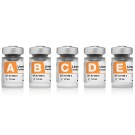Linearity FD Cardiac Markers

Order Number: K710M-5
25.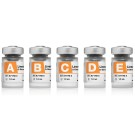Linearity FD D-Dimer

Order Number: K717M-5
26.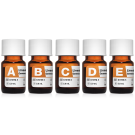Linearity FD General Chemistry

Order Number: K701M-5
27.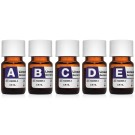Linearity FD General Chemistry Panel 1 for Abbott Systems

Order Number: K940M-5
28.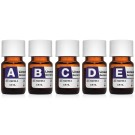Linearity FD General Chemistry Panel 2 for Abbott Systems

Order Number: K941M-5
29.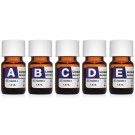Linearity FD General Chemistry Panel 3 for Abbott Systems

Order Number: K942M-5
30.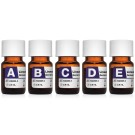Linearity FD General Chemistry Panel 4 for Abbott Systems

Order Number: K943M-5
1. 1
2. 2